# c#使用泛型实现一个自己的list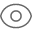369人阅读 2019/9/27 10:54 总访问：204899 评论:0 手机 收藏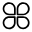分类: .NET

```static void Main(string[] args)
{
MyList<int> intlist = new MyList<int>();
for (int i = 0; i < 8; i++)
{
}
//删除
intlist.Remove(0);
Console.WriteLine("条数:" + intlist.Count);
Console.WriteLine("容量:" + intlist.Capacity);
//遍历
for (int i = 0; i < intlist.Count; i++)
{
Console.WriteLine("item:" + intlist[i]);
}
//foreach遍历
foreach (int item in intlist)
{
Console.WriteLine(item);
}
}```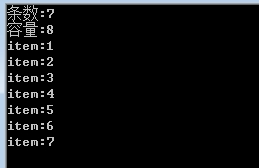```public void Add(T t)
{
//当装不下的时候扩容
int length = array.Length;
if (index == length)
{
//创建一个新数组
T[] temp = new T[length * 2];
Array.Copy(array, temp, length);
array = temp;
}
array[index] = t;
index++;
}```

```    //list条数
public int Count
{
get
{
return index;
}
}
//list容量
public int Capacity
{
get
{
return array.Length;
}
}```

• 方法1：创建一个新的数组，然后把除了删除的元素复制到新数组去，也就是跳过需要删除的元素即可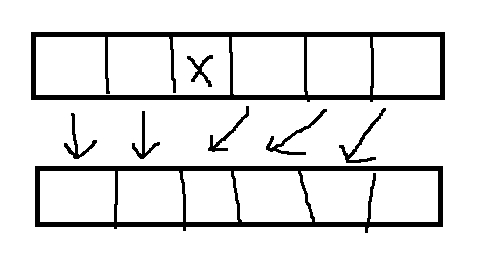```public void Remove(T t)
{
//需要删除的位置
int poi = -1;
for (int i = 0; i < Count; i++)
{
if (t.Equals(array[i]))
{
poi = i;
break;
}
}
if (poi == -1)
{
return;
}
//创建一个新数组
T[] temp = new T[array.Length];
for (int i = 0, j = 0; i < array.Length - 1; i++, j++)
{
//这个刚好是需要被删除
if (i == poi)
{
j++;
}
temp[i] = array[j];
}
array = temp;
index--;
}```
• 方法2：其实没有必要创建一个新的数组，我们可以在当前这个数组上进行位置的改动，删除位置前不需要动后面的位置依次向前移动即可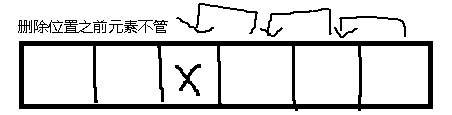代码的话很简单一个Array.Copy即可搞定

```public void Remove(T t)
{
//找到需要删除的位置
var poi = FindIndex(t);
//源数组，源数组开始位置 。 目标数组，目标数组开始位置，循环赋值次数
Array.Copy(array, poi + 1, array, poi, index - poi - 1);
//数组长度减一,因为删除了长度会减少一
index--;
}```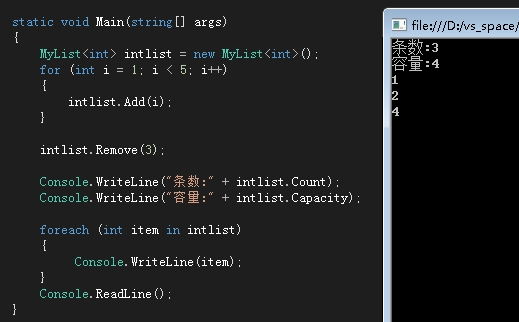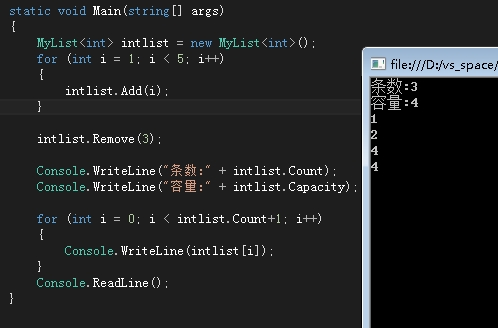```public T this[int _index]
{
get
{
if (_index >= index)
{
throw new Exception("索引超出了长度");
}
return array[_index];
}
set
{
array[index] = value;
index++;
}
}```

```static void Main(string[] args)
{
List<int> intlist = new List<int>();
for (int i = 1; i < 5; i++)
{
}
intlist.Remove(3);
//反射获取list中私有字段
var result = intlist.GetType().GetFields(BindingFlags.NonPublic | BindingFlags.Instance);
int[] findArray = null;
foreach (var item in result)
{
//找到list中维护的数组,不要问我怎么找到的,遍历看名字就知道了
if (item.Name == "_items")
{
findArray = (int[])item.GetValue(intlist);
}
}
foreach (int item in findArray)
{
Console.WriteLine(item);
}
}```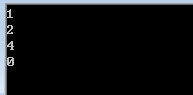微软果然严谨这种问题都处理了，微软估计是赋予了这个泛型的默认值，ok跟着大佬的步伐我们也去这样处理一下

```public void Remove(T t)
{
var poi = FindIndex(t);
Array.Copy(array, poi + 1, array, poi, index - poi - 1);
//处理移动最后一个的元素为该类型的默认值
array[index-1] = default(T);
index--;
}```

array[index-1] = default(T);

ok我们把list换成我们自己的mylist试试，因为我自己写的list，里边维护的数组叫array所以也要修改一下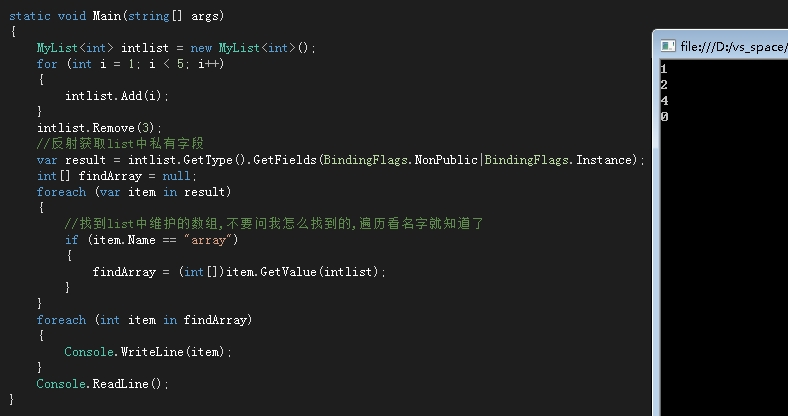ok搞定研究点这种问题还是有点意思

## {{titleitem}} {{titleitem}}

{{item.content}}

{{item.articleTitle}}
{{item.blogName}} : {{item.content}}
ICP备案 ：渝ICP备18016597号-1